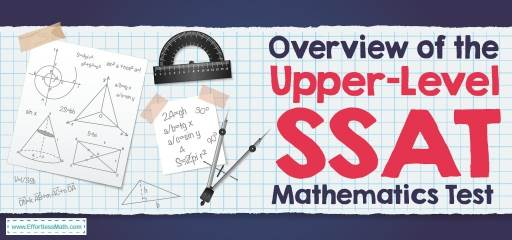# Overview of Upper-Level SSAT Mathematics TestThe High School Admission Test is as known as SSAT is used for admission to private middle schools and high schools. If you want to attend an elite private middle school or high school, this is a test you should do well.

The upper-Level SSAT is a multiple-choice test for students currently in grades 8-11 that involves the quantitative or mathematics, verbal, and reading comprehension sections plus a non-graded writing sample. This test consists of 167 questions and you have 3 hours and 5 minutes to complete all questions.

## How is the structure of the math section of Upper-Level SSAT?

The Upper-Level SSAT Quantitative or Math section has 50 questions. The math section is split into two, with 25 five-choice multiple-choice questions in each. You will be given 30 minutes to complete each of the Quantitative or Math sections. To answer each question, you have a little over a minute.

The Upper-Level SSAT math measures your ability to solve problems involving arithmetic, elementary algebra, geometry, and other concepts. These topics involve:

### Number Concepts and Operations

• Arithmetic word problems (including percent, ratio)
• Basic concepts of division, subtraction, addition, and multiplication
• Rational numbers
• Frequencies
• Sequences and series
• Estimation

### Elementary Algebra

• Algebraic word problems
• Properties of exponents
• Equations of lines
• Absolute value
• Patterns

### Geometry/Measurement

• Area and perimeter of a polygon
• Area and circumference of a circle
• The volume of a cube, cylinder, box
• Properties of parallel and perpendicular lines
• Properties of isosceles, right, equilateral triangles, and Pythagorean theory
• Slope
• Coordinate geometry

### Data analysis/probability

• Interpretation (tables, graphs)
• Probability
• Trends and inferences

Many of the questions that appear in the quantitative sections of the Upper-Level SSAT are mathematically structured and directly state what you need to do, others may not. In these problems, carefully consider how to ask the question and how to provide information to determine the operation (s) to be performed.

## IstheUpper-Level SSAT mathematics hard?

The questions in math sections of SSAT will progress in difficulty as the student works his or her way through the section. Because the student loses ¼ points for each incorrect answer, the student should not make random guesses in the SSAT math sections.

## Can you use the calculator on the Upper-Level SSAT mathematics?

Students are not allowed to use the calculator in SSAT mathematics.

## Do you get a formula sheet on the Upper-Level SSAT mathematics?

The SSAT Upper Level does not provide a list of all the basic formulas needed to know the test. It means that students should be able to memorize many mathematical formulas at an SSAT Upper Level.

## How is the Upper-Level SSAT test scored?

Your score report is divided into sections. Each section gives a high score of 800 and a low of 500. You also be given a total scaled score. The lowest possible score is 1500, and the highest is 2400.

## The Best Books to Ace the SSAT Upper-Level MathTest

### What people say about "Overview of Upper-Level SSAT Mathematics Test - Effortless Math: We Help Students Learn to LOVE Mathematics"?

No one replied yet.

X
52% OFF

Limited time only!

Save Over 52%

SAVE \$40

It was \$76.99 now it is \$36.99